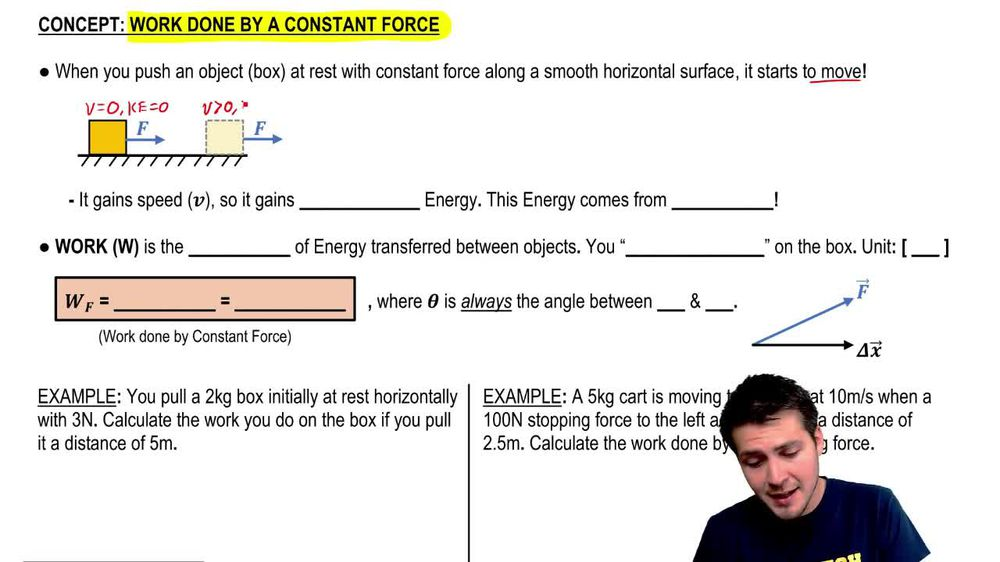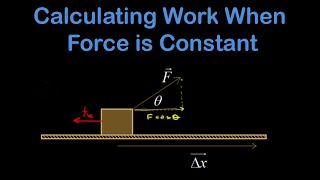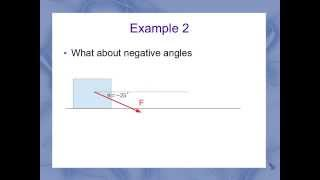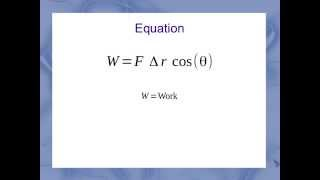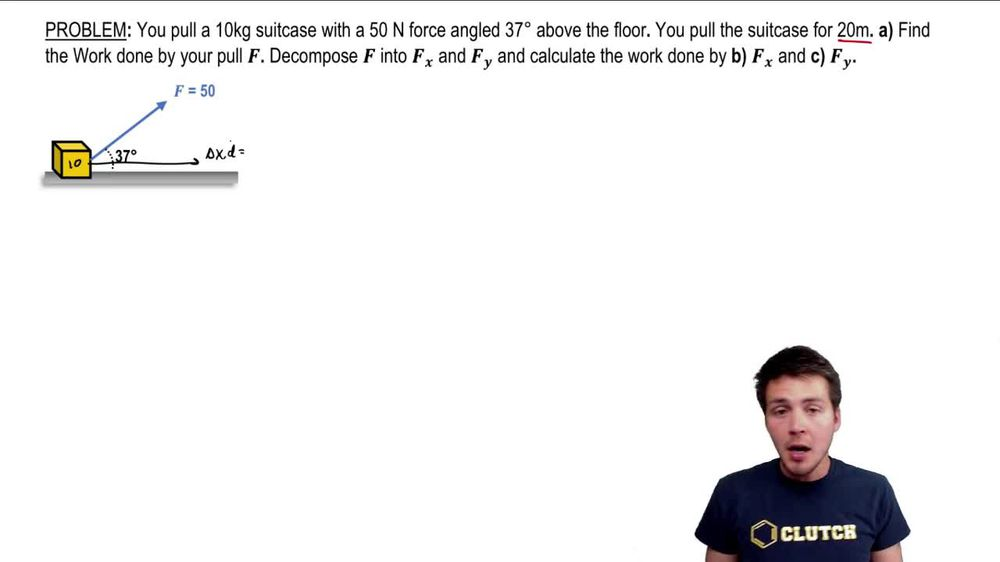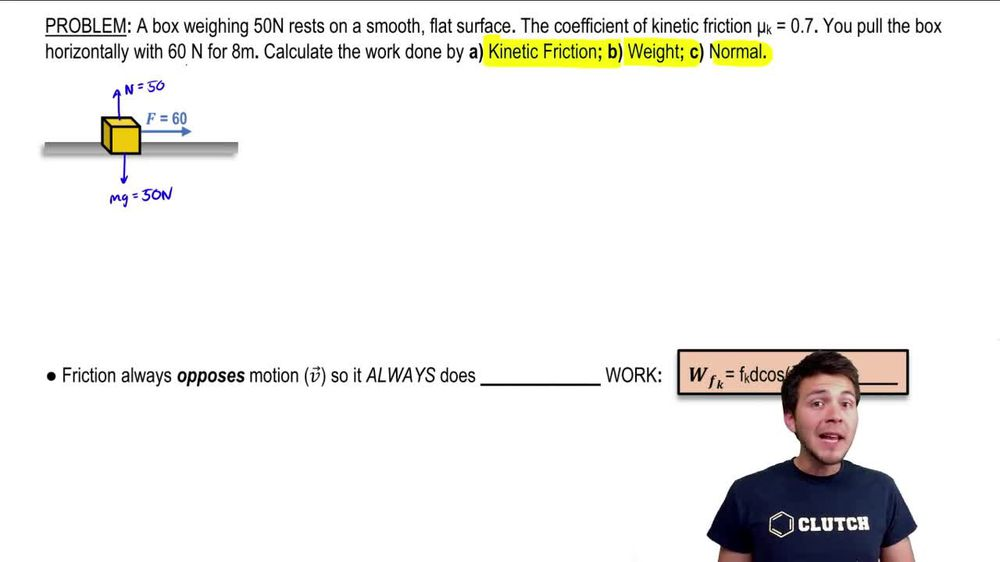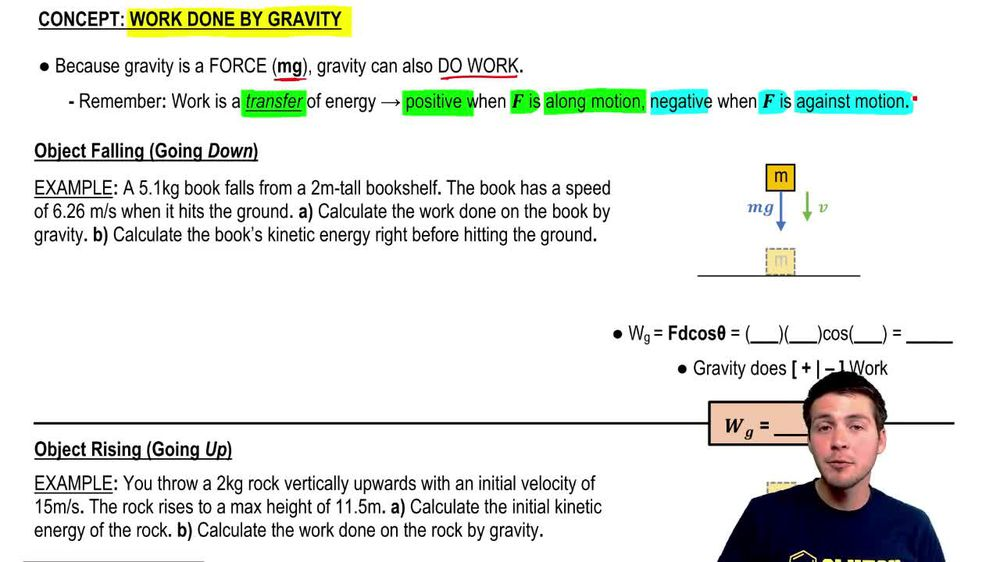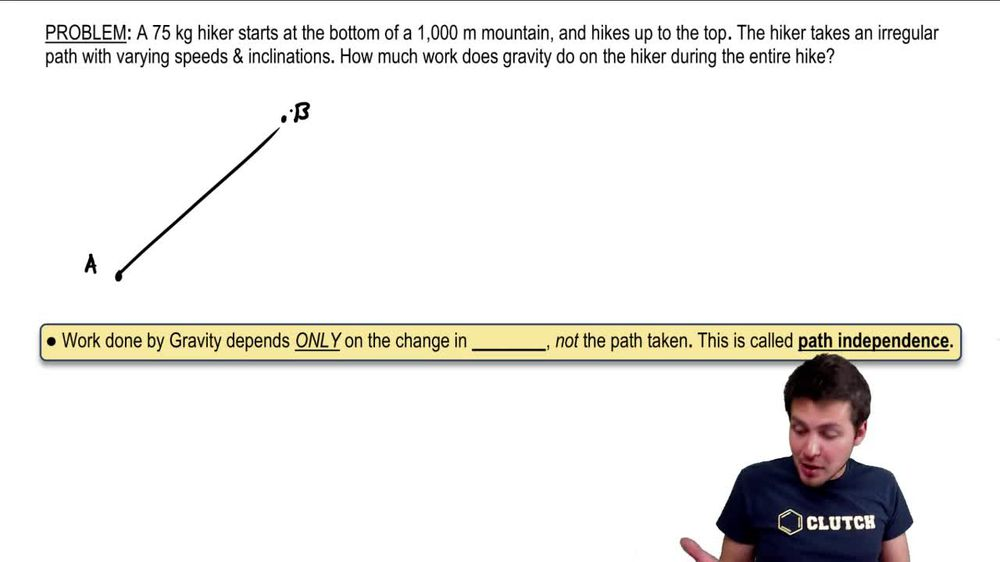Start typing, then use the up and down arrows to select an option from the list.
1. 9. Work & Energy2. Intro to Calculating Work# Work Done on Curvy (Not straight) Paths

by Patrick Ford
219 views
1
1
1
All right guys, let's check out this problem here, we have a hiker who starts at the bottom of the mountain. So basically I'm gonna call this point A. Like this and they're trying to get to the top of this mountain, so I'm gonna call this point B. But instead of taking a straight path like up like this and therefore kind of looking at an inclined plane, what's actually happening is this hiker is going to take an irregular path with varying speeds and also inclination. So what does it look like? It's kind of like a squiggly path to get up to the top here of this mountain. We want to do is we want to figure out the work that is done by gravity on the hiker during this entire hike. So basically that's what I'm going to draw a little line like this and we know that this height here of the mountain, this is going to be my delta Y. Is going to be 1000 m. But the path that I'm taking from A to B is actually going to be sort of not straight, it's gonna be curvy like this. So how do I calculate the work that's done by gravity? Well remember that the equation is just negative MG. Times delta Y. And we have to do is we have to figure out a direction first of positive, So because I'm going from the bottom to the top, I'm going to call the upward direction positive like this. So what this means is that our delta Y. Is actually positive 1000 here. So if we get to the equation W. G equals negative MG delta. Why we run into a problem here? Because you might think that as we go along this path here, the work that's done is going to be sort of changing and you're actually right, it is at any point along the path right here for this hiker, we know that the MG is gonna point downwards. However, what are the really cool things about the work that's done by gravity is from the equation, we can tell that the work done only really just depends on delta, why it's only depending on the change in height here. So the work that's done by gravity depends only on the change in your heights. And it doesn't actually cause it doesn't actually depend on the path that's taken. So you wouldn't be able to solve this problem if you didn't know this. So this is actually called path independence. You might see that in your textbooks. It basically just means that it doesn't matter what path you take. All that matters is that you started today and ended up B and the difference between those two is m. So the work done by gravity is just gonna be negative. We have 75 times 9.8 And then we're gonna multiply this by positive 1000. So as we as we should expect to work done by gravity is gonna be negative and it's going to be 735,000 jewels. That's the work that is done by gravity. Alright, so again, only just depends on the height. It doesn't actually matter the path that you take here. We'll talk about that more in a later video. So that's it for this one guys.